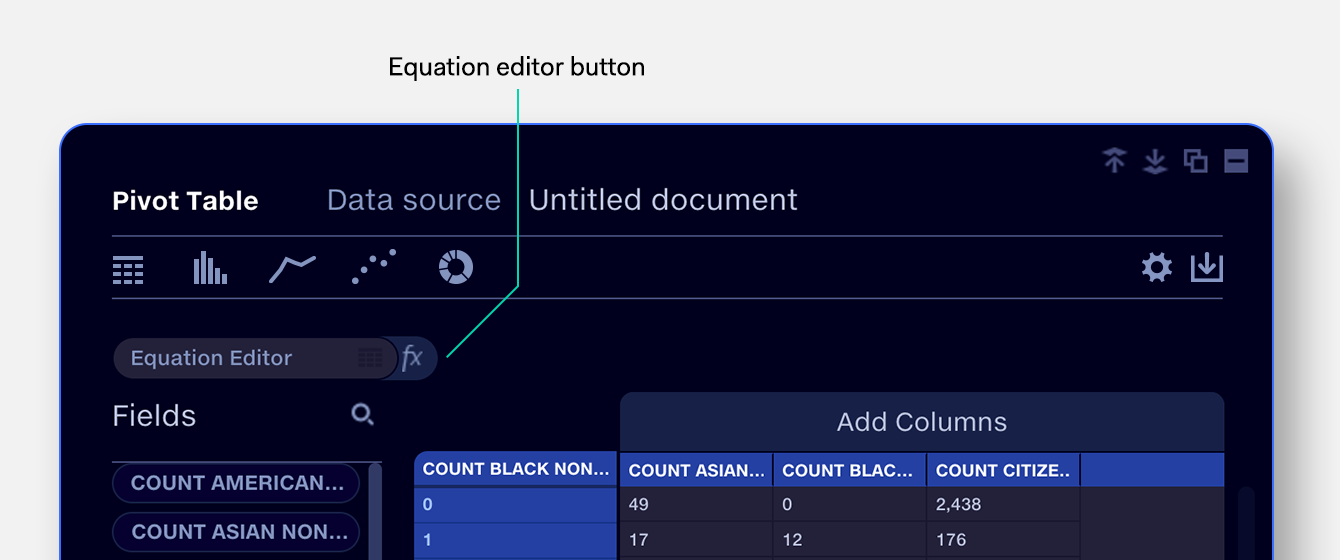Equation Editor
Using the editorThe equation editor is a pop-out formula bar in the pivot frame which allows you to perform on-the-fly calculations with your source data, e.g. Loss Ratio = ‘Claims’ / ‘Premium’.
Create a calculated field
1.
Create a pivot and select a data source.

2.
Click on the fx button. This opens the formula bar and creates a calculated field
in the list below.

3.
Name the calculated field. The new name is reflected in the list below.
Calculated fields are marked with the fx icon.

4.
Enter a field or function in the formula bar to the right. Starting to type will suggest:

a.
from the fields in your data source;

b.
from the library of functions. In the drop-down list, functions are marked with the fx symbol.

5.
Enter operators or fields to complete the equation. Be sure to follow the correct   syntax.

6.

7.
Drag the new calculated field into the pivot table to view the results.

8.
Once the calculated field produces the expected results, the formula bar can be closed
by clicking the fx button. This will reset the equation, ready for a new calculated field to
be created.
You may see a result ‘NaN’ (‘Not a number’), or ‘∞’ if performing unresolveable equations such as 0/0.
Tip
Press tab to move between the calculated field name
and the formula bar
Editing calculated fields
To edit or rename a calculated field:

1.
Locate the calculated field in the fields list. Calculated fields are marked with the fx icon.

2.
Drag the calculated field into the equation editor.
The formula bar expands to show the equation.

3.
Edit the name or equation.
Delete a calculated field
1.
Hover the calculated field in the fields list. The fx icon changes to a minus symbol [-].

2.
Click the minus symbol [-]